# Mensuration circle

## Mensuration of Class 8

### CIRCLE: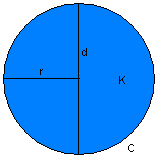d = 2r diameterradius C = 2πr circumference >radius
 C = π× d circumferencediametercircumferencearea
 K = πr2 arearadiusareadiameterareacircumferenceradius

### SEGMENT OF A CIRCLE: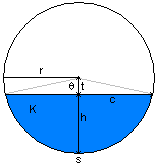chord lengthcircle radiuscircle center to chord midpoint distancesegment areacircle radiuscentral angle s = θ× r arc lengthcircle radius
 h = r -t segment height r = h + t circle radius t = r – h circle center to chord midpoint distance

### SECTOR OF A CIRCLE:

A sector of a circle is a pie-shaped region bounded by an arc and an angle.
On this page we'll show you how to find the arc length a and the area A of the sector.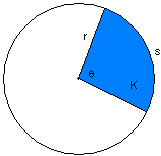sector areacircle radiuscentral angle

### ARC OF A CIRCLE: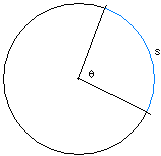S = r ×θ arc lengthcircle radiuscentral angle

We'll use a circle with radius 15 cm and central angle 60°.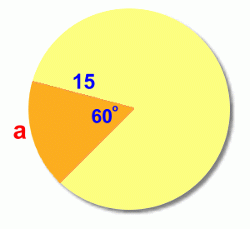The first step is to find out what fraction of the circleis represented by the central angle 60°:The sector represents 1/6 of the circle,or 0.1666... of the circle.

To find the arc length a, we now need to find the circumference (the distance around the full circle), and then find 1/6 of it (multiply it by 0.1666...)

This is the length of the arc a.

C = 2πr

= 2π⋅ 15

= 94.248

a =⋅ 94.248 cm

= 15.7 cm

This method will work for any sector in any circle. You just need to find the fraction of the circle that the sector angle represents, and then find that fraction of the circumference.Here's a quicker formula to use, that summarizes what we did in one calculation.

### SOLVED EXAMPLES

question 1.Find the circumference and area of a circle of radius 6.5 cm.

Solution: Here r = 6.5 cm

Circumference of the circlequestion 2.A wire is bent into the form of a square of side 30 cm. The wire is straightened and bent into the form of a circle. What will be the radius of the circle so formed? (Take π = 22/7)

Solution:Length of wire = Perimeter of the square = (30 × 4) cm = 120 cm.

When this wire is bent into the form of a circle, then clearly the circumference of the circle would be 120 cm.

Let r be the radius of this circle.

Then, circumferencer = 19.09 cm

## CBSE NCERT Solutions for Class 8 Maths

### Notes,worksheet and solved question for Maths class 8

Check your marks in a chapter which you have complited in school from Physics Wallah chapter wise online test just click on the link given below